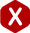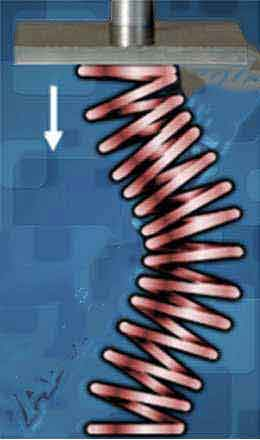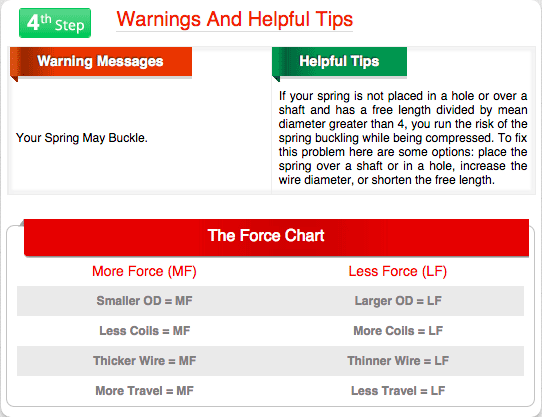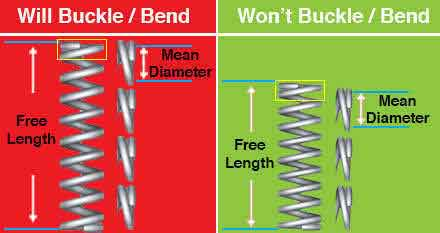<# Coil Springs Slenderness Ratio

#### Coil Spring Slenderness Ratio

##### Definition: It is the proportional ratio between the diameter of a spring’s coils and the free length. It defines the slenderness of your spring thus determining if your spring will buckle or bend when being compressed

Spring stability is something you want to achieve when creating a coil spring design. This is why all factors must be taken into note, including the coil spring’s slenderness ratio. Unless your coil spring is going over a shaft or inside a hole, you want to make sure that the spring will not bend or buckle when it is being compressed. Spring bending or buckling can cause your spring to be ejected from the device. If you place the spring over a shaft or inside a hole, there might be some friction between the spring’s diameter and the walls of the shaft or hole. In this case, you might need a little bit more load to compensate for that friction which would prevent the spring to travel freely. Nevertheless, placing the spring inside a hole or over a shaft is the best option if you have a high coil spring slenderness ratio.

#### How It Works

Coil spring slenderness ratio is based on the proportion of such spring's dimensions. If the free length of your spring is too long in comparison to the spring’s diameter, the spring is likely to buckle. To calculate the coil spring slenderness ratio you must calculate mean diameter first. You do this by either adding one wire diameter to the inner diameter or subtracting one wire diameter from the outer diameter. Then, you must divide the spring’s free length by the mean diameter as shown in the formulas.

##### Calculate Mean Diameter
Outer Diameter – Wire Diameter = Mean Diameter
OD – WD = MD

Inner Diameter + Wire Diameter = Mean Diameter
ID + WD = MD

Note: If your coil spring’s slenderness ratio is over 4, you run the risk of the spring bending or buckling.

#### Coil Spring Buckling/Bending#### Use Spring Creator

You may determine whether your spring will buckle by using our coil spring calculator, Spring Creator. Spring Creator provides you with warnings and helpful tips regarding your spring design. One of these warnings is “Your Spring May Buckle”, shown to the right. The helpful tip advises that you either place the spring over a shaft or inside a hole, increase the wire diameter, or shorten the free length. You may also increase the outer diameter. The way to know just how much you should adjust your spring’s dimensions is by inversing the equation which helped you calculate your coil spring’s slenderness ratio.

In the case that you may want to adjust your spring’s free length, you will inverse the coil spring equation for slenderness ratio so that, to the right of the equal sign, you will have the free length as an unknown. To the left of the equal sign, you will be multiplying the desired coil spring slenderness ratio (in this case we’ll use 3.9 which is right below 4) by the mean diameter (we’ll use 0.462”).#### Make Adjustments to Avoid Buckling

Slenderness Ratio x Mean Diameter = Free Length
SR x MD = FL
3.9 x 0.462 = 1.8018

To double-check the above, you may input all the values into the coil spring's slenderness ratio formula into their respective order.

FL ÷ MD = SR
1.8018 ÷ 0.462 = SR
3.9 = SR

#### Slenderness Ratio Examples

The diagram below explains the proportional ratio between the spring's mean diameter and the free length. The spring to the left is too long and its length is four times the mean diameter thus having a high slenderness ratio which will cause it to bend or buckle. The spring on the right has a free length that is three times the size of the mean diameter. This means that we're not exceeding the maximum of 4 to 1.